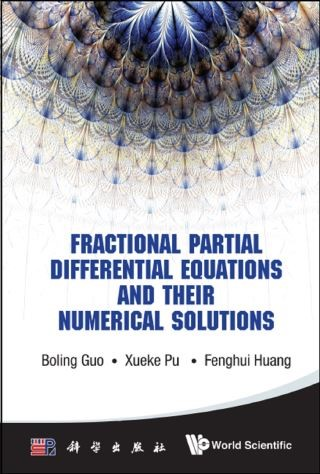## Fractional Partial Differential Equations And Their Numerical Solutions• Description
• Author
• Info
• Reviews

### Description

This book aims to introduce some new trends and results on the study of the fractional differential equations, and to provide a good understanding of this field to beginners who are interested in this field, which is the authors' beautiful hope.

This book describes theoretical and numerical aspects of the fractional partial differential equations, including the authors' researches in this field, such as the fractional Nonlinear Schrödinger equations, fractional Landau–Lifshitz equations and fractional Ginzburg–Landau equations. It also covers enough fundamental knowledge on the fractional derivatives and fractional integrals, and enough background of the fractional PDEs.

Contents:
• Physics Background
• Fractional Calculus and Fractional Differential Equations
• Fractional Partial Differential Equations
• Numerical Approximations in Fractional Calculus
• Numerical Methods for the Fractional Ordinary Differential Equations
• Numerical Methods for Fractional Partial Differential Equations

Readership: Graduate students and researchers in mathematical physics, numerical analysis and computational mathematics.
Key Features:
• This book covers the fundamentals of this field, especially for the beginners
• The book covers new trends and results in this field
• The book covers numerical results, which will be of broad interests to researchers